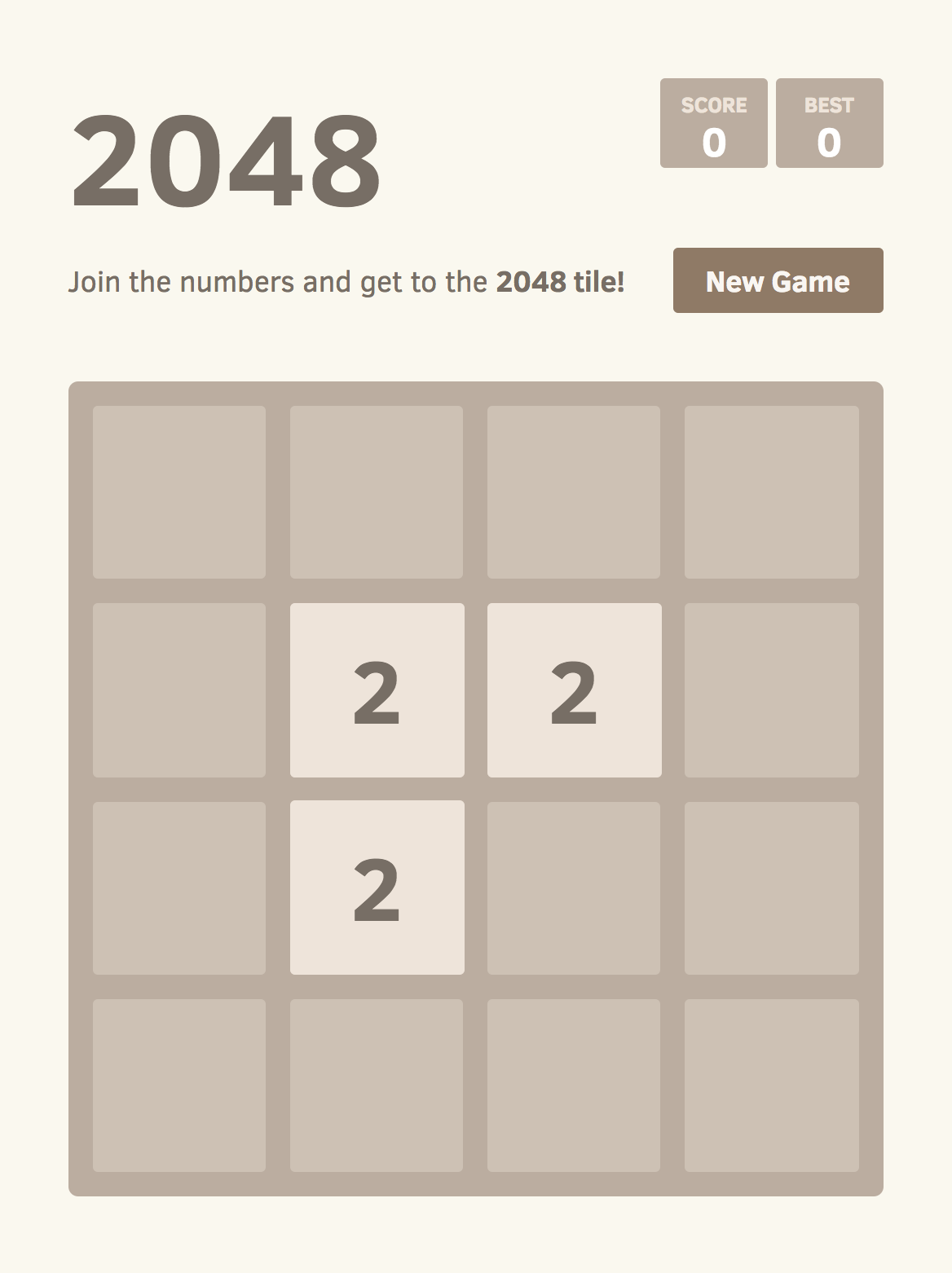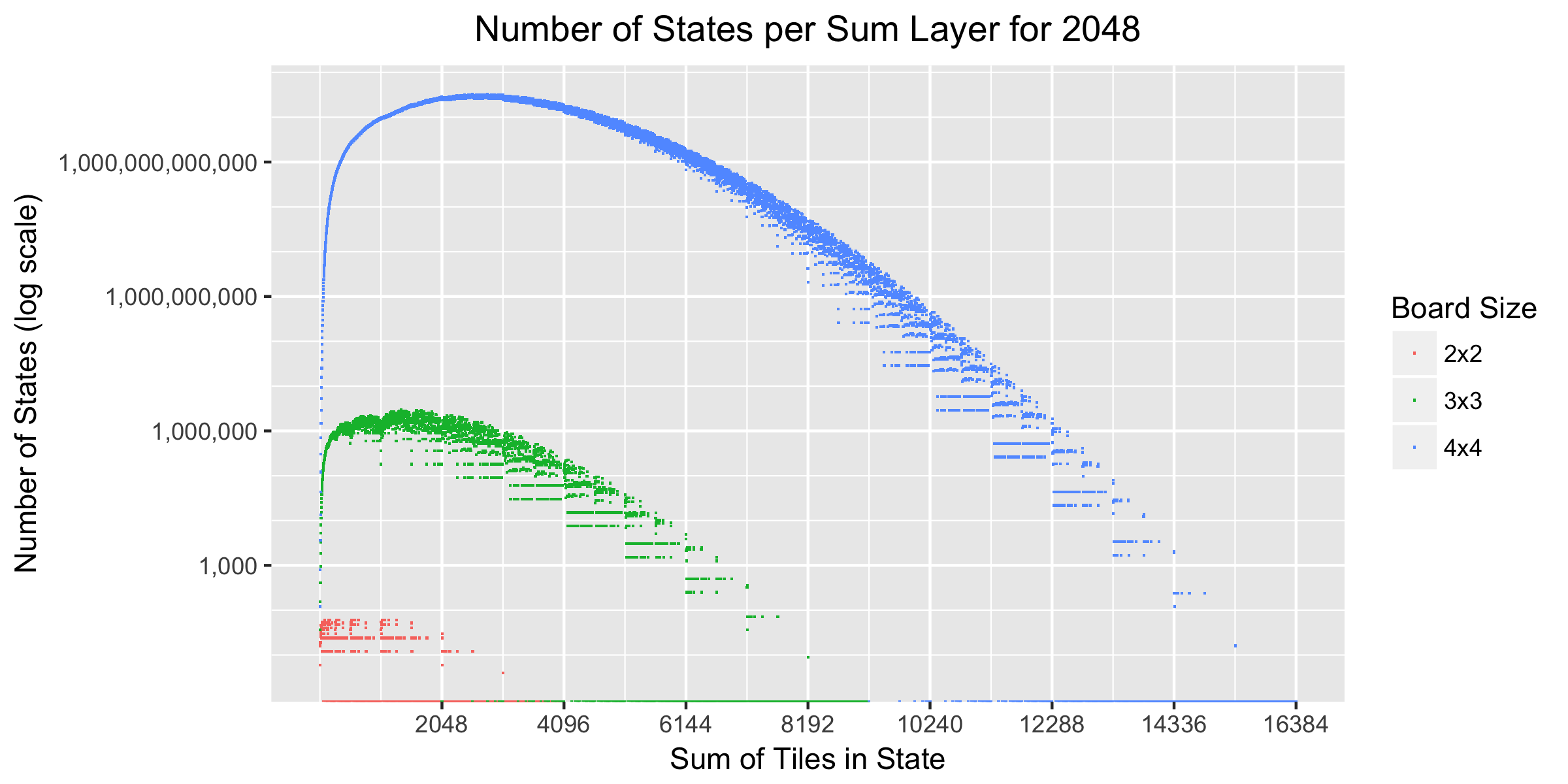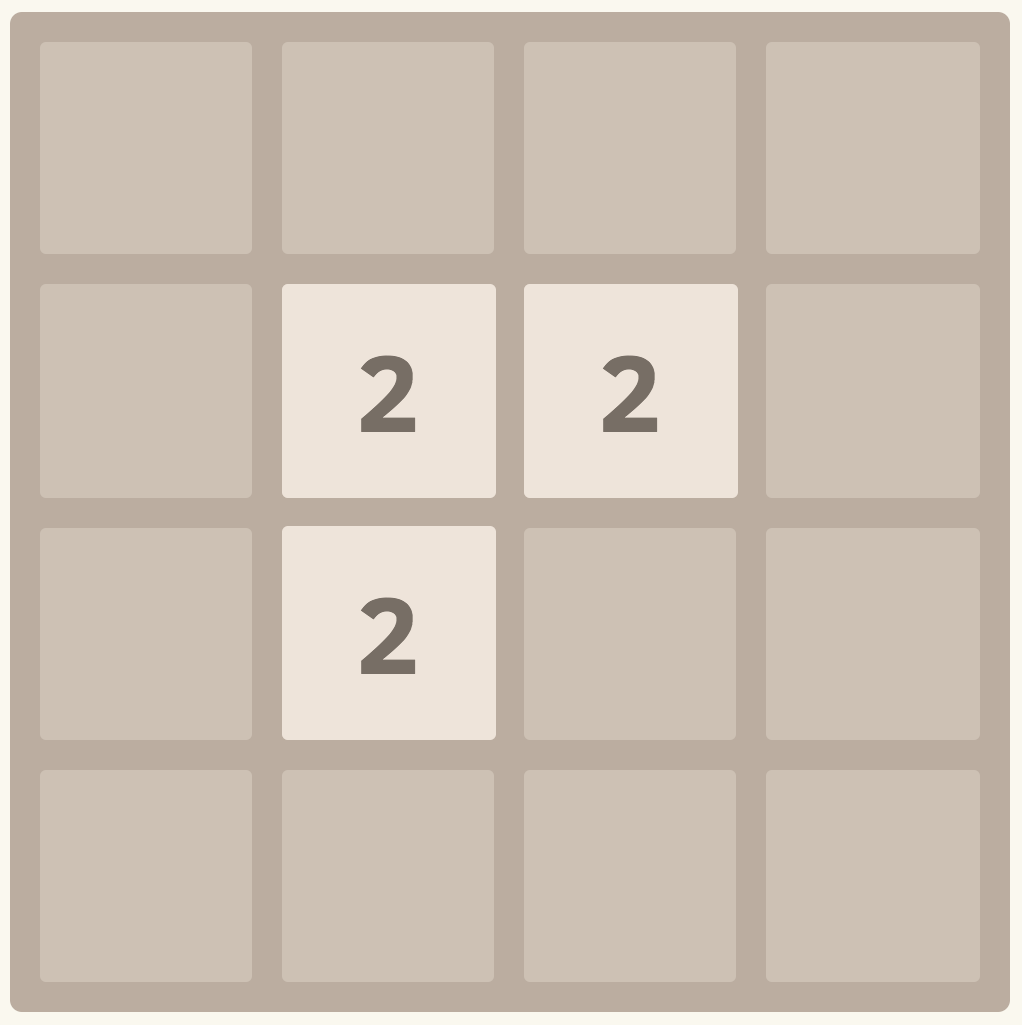This post is the second in a series. Next: Counting States by Exhaustive Enumeration.In my last 2048 post, I found that it takes at least 938.8 moves on average to win a game of 2048. The main simplification that enabled that calculation was to ignore the structure of the board — essentially to throw the tiles into a bag instead of placing them on a board. With the ‘bag’ simplification, we were able to model the game as a Markov chain with only 3486 distinct states.

In this post, we’ll make a first cut at counting the number of states without the bag simplification. That is, in this post a state captures the complete configuration of the board by specifying which tile, if any, is in each of the board’s cells. We would therefore expect there to be a lot more states of this kind, now that the positions of the tiles (and cells without tiles) are included, and we will see that this is indeed the case.

To do so, we will use some (simple) techniques from enumerative combinatorics to exclude some states that we can write down but which can’t actually occur in the game, such as the one above. The results will also apply to 2048-like games played on different boards (not just 4x4) and up to different tiles (not just the 2048 tile). We’ll see that such games on smaller boards and/or to smaller tiles have far fewer states than the full 4x4 game to 2048, and that the techniques used here are relatively much more effective at reducing the estimated number of states when the board size is small. As a bonus, we’ll also see that the 4x4 board is the smallest square board on which it is possible to reach the 2048 tile.

# Baseline

The most straightforward way to estimate the number of states in 2048 is to observe that there are 16 cells, and each cell can either be blank or contain a tile with a value that is one of the 11 powers of 2 from 2 to 2048. That gives 12 possibilities for each of the 16 cells, for a total of $$12^{16}$$, or 184 quadrillion (~$$10^{17}$$), possible states that we can write in this way. For comparison, some estimates put the number of possible board configurations for the game of chess at around $$10^{45}$$ states, and the latest estimates for the game of Go are around $$10^{170}$$ states, so while $$10^{17}$$ is large, it’s certainly not the largest as games go.

For 2048-like games more generally, let $$B$$ be the board size, and let $$K$$ be the exponent of the winning tile with value $$2^K$$. For convenience, let $$C$$ denote the number of cells on the board, so $$C=B^2$$. For the usual 4x4 game to 2048, $$B=4$$, $$C=16$$, and $$K = 11$$, since $$2^{11} = 2048$$, and our estimate for the number of states is $(K + 1)^C.$ Now let’s see how we can refine this estimate.

First, since the game ends when we obtain a $$2^K$$ tile, we don’t particularly care about where that tile is or what else is on the board. We can therefore condense all of the states with a $$2^K$$ tile into a special “win” state. In the remaining states, each cell can either be blank or hold one of $$K - 1$$ tiles. This reduces the number of states we have to worry about to $K^C + 1$ where the $$1$$ is for the win state.

Second, we can observe that some of those $$K^C$$ states can never occur in the game. In particular, the rules of the game imply two useful properties:

Property 1: There are always at least two tiles on the board.

Property 2: There is always at least one 2 or 4 tile on the board.

The first property holds because even if you start with two tiles and merge them, there is still one left, and then the game adds a random tile, leaving two tiles. The second property holds because the game always adds a 2 or 4 tile after each move.

We therefore know that in any valid state there must be at least two tiles on the board, and that one of them must be a 2 or 4 tile. To account for this, we can subtract all states with no 2 or 4 tile, of which are $$(K-2)^C$$, and also the states with just one 2 tile and all other cells empty, of which there are $$C$$, and the states with only one 4 tile and all other cells empty, of which there are again $$C$$. This gives an estimate of $K^C - (K-2)^C - 2C + 1$ states in total. Of course, when $$K$$ or $$C$$ is large, this looks pretty much just like $$K^C$$, which is the dominant term, but this correction is more significant for smaller values.

Let’s use this formula to tabulate the estimated number of states various board sizes and maximum tiles:

Maximum Tile Board Size
2x2 3x3 4x4
8 73 19,665 43,046,689
16 233 261,615 4,294,901,729
32 537 1,933,425 152,544,843,873
64 1,033 9,815,535 2,816,814,940,129
128 1,769 38,400,465 33,080,342,678,945
256 2,793 124,140,015 278,653,866,803,169
512 4,153 347,066,865 1,819,787,258,282,209
1024 5,897 865,782,255 9,718,525,023,289,313
2048 8,073 1,970,527,185 44,096,709,674,720,289

We can see immediately that the 2x2 and 3x3 games have many orders of magnitude fewer states than the 4x4 game. We’ve also also managed to reduce our estimate for the number of tiles in the 4x4 game to 2048 to “only” 44 quadrillion, or ~$$10^{16}$$.

# Counting in Layers

To gain some additional insight into these state counts, we can take advantage of another important property, which was also useful in the last post:

Property 3: The sum of the tiles on the board increases by either 2 or 4 with each move.

This holds because merging two tiles does not change the sum of the tiles on the board, and the game then adds either a 2 or a 4 tile.

Property 3 implies that states never repeat in the course of a game. This means that we can organize the states into layers according to the sum of their tiles. If the game is in a state in the layer with sum 10, we know that the next state must be in the layer with either sum 12 or sum 14. It turns out we can also count the number of states in each layer, as follows.

Let $$S$$ denote the sum of the tiles on the board. We want to count the number of ways that up to $$C$$ numbers, each of which is a power of 2 between 2 and $$2^{K-1}$$, can be added together to produce $$S$$.

Fortunately, this turns out to be a variation on a well-studied problem in combinatorics: counting the compositions of an integer. In general, a composition of an integer $$S$$ is an ordered collection of integers that sum to $$S$$; each integer in the collection is called a part. For example, there are four compositions of the integer $$3$$, namely $$1 + 1 + 1$$, $$1 + 2$$, $$2 + 1$$ and $$3$$. When there are restrictions on the parts, such as being a power of two and only having a certain number of parts, the term is a restricted composition.

Even more fortunately, Chinn and Niederhausen (2004) 1 have already studied exactly this kind of restricted composition and derived a recurrence that allows us count the number of compositions in which there are a specific number of parts, and each part is a power of 2. Let $$N(s, c)$$ denote the number of compositions of a (positive) integer $$s$$ into exactly $$c$$ parts where each part is a power of 2. It then holds that $N(s, c) = \begin{cases} \sum_{i = 0}^{\lfloor \log_2 s \rfloor} N(s - 2^i, c - 1), & 2 \le c \le s \\\ 1, & c = 1 \textrm{ and } s \textrm{ is a power of 2} \\\ 0, & \textrm{otherwise} \end{cases}$ because for every composition of $$s - 2^i$$ into $$c - 1$$ parts, we can obtain a composition of $$s$$ with $$c$$ parts by adding one part with value $$2^i$$.

We now just need to make a few minor adjustments to the summation bounds: we would like to use powers of 2 starting at 2 and at most $$2^{K-1}$$, since if we have a $$2^K$$ tile the game is won. To this end, let $$N_m(s, c)$$ denote the number of compositions of $$s$$ into exactly $$c$$ parts where each part is a power of 2 between $$2^m$$ and $$2^{K-1}$$. This is given by $N_m(s, c) = \begin{cases} \sum_{i = m}^{K - 1} N(s - 2^i, c - 1), & 2 \le c \le s \\\ 1, & c = 1 \textrm{ and } s = 2^i \textrm{ for some } i \in \{ m, \ldots, K-1 \} \\\ 0, & \textrm{otherwise} \end{cases}$ following the same logic as above.

Now we have a formula for exactly $$c$$ parts, but we want a formula for up to $$c$$ parts. We can follow the same rationale as in the previous section: subtract off the states with no 2 or 4 tile, of which there are $$N_3(s, c)$$. According to property 1, we need at least 2 parts, so we start summing at $$c=2$$. This gives $\sum_{c = 2}^{C} {C \choose c} \left( N_1(s, c) - N_3(s, c) \right)$ as our estimate for the number of states with sum $$s$$. Here $$C \choose c$$ is a binomial coefficient that gives the number ways of choosing $$c$$ of the possible $$C$$ cells into which to place the tiles. Let’s plot it out.In terms of magnitude, we can see that the 2x2 game never has more than 60 states in any layer, the 3x3 game peaks at about 3 million states per layer, and the 4x4 game peaks at about 32 trillion ($$10^{13}$$) states per layer. The number of states grows rapidly early in the game but then tapers off and eventually decreases as the board fills up. On the decreasing portion of the curve, we see discontinuities: particularly for higher sums, it may happen that there are no tiles that will fit on the board and sum to that value.

The upper limit on the horizontal axis arises because we can have $$C$$ values, each up to $$2^{K-1}$$, so the maximum achievable sum is $$C 2^{K-1}$$, or 16,384 for the 4x4 game to 2048.

Finally, it’s worth noting that if we sum the number of states in each layer over all of the possible layer sums from 4 to $$C 2^{K-1}$$, and add one for the special win state, we get the same number of states as we estimated in the previous section, which is a helpful sanity check.

### Layer Reachability

Another useful consequence of Property 3 is that if two consecutive layers have no states, it’s not possible to reach later layers. This is because the sum can increase by at most 4 per turn; if there are two adjacent layers with no states, then the sum would have to increase by 6 in a single move in order to ‘jump’ to the subsequent layer, which is not possible. Finding the layer sums that contain no states according to the calculation above therefore allows us to tighten up our estimate by excluding states in unreachable layers after the last reachable layer. The largest reachable layer sums (without ever attaining a 2048 tile) are:

Board Size Largest Reachable Layer Sum
2x2 60
3x3 2,044
4x4 9,212

This table also tells us that the highest tile we can reach on the 2x2 board is the 32 tile, because the 64 tile can’t occur in a layer with sum 60 or less, and similarly highest reachable tile on the 3x3 board is the 1024 tile. This means that the 4x4 board is the smallest square board on which it’s possible to reach the 2048 tile 2. For the 4x4 board, the largest layer sum we can reach without reaching a 2048 tile (and therefore winning) is 9,212, but larger sums would be reachable if we did allow a 2048 tile.

Taking into account layer reachability, the new estimates for the number of states are:

Maximum Tile Method Board Size
2x2 3x3 4x4
8 Baseline 7319,66543,046,689
Layer Reachability 7319,66543,046,689
16 Baseline 233261,6154,294,901,729
Layer Reachability 233261,6154,294,901,729
32 Baseline 5371,933,425152,544,843,873
Layer Reachability 5291,933,407152,544,843,841
64 Baseline 1,0339,815,5352,816,814,940,129
Layer Reachability 9059,814,4372,816,814,934,817
128 Baseline 1,76938,400,46533,080,342,678,945
Layer Reachability 90538,369,57133,080,342,314,753
256 Baseline 2,793124,140,015278,653,866,803,169
Layer Reachability 905123,560,373278,653,849,430,401
512 Baseline 4,153347,066,8651,819,787,258,282,209
Layer Reachability 905339,166,4851,819,786,604,950,209
1024 Baseline 5,897865,782,2559,718,525,023,289,313
Layer Reachability 905786,513,8199,718,504,608,259,073
2048 Baseline 8,0731,970,527,18544,096,709,674,720,289
Layer Reachability 9051,400,665,57544,096,167,159,459,777

This has a large effect on the 2x2 board, reducing the number of states from 8,073 to 905 for the game up to 2048, and it’s notable that the figure for the number of reachable states does not increase from 905 for maximum tiles over 32, because it’s not possible to reach tiles larger than 32 on a 2x2 board. It also has some effect on the 3x3 board, but on the 4x4 board, there is relatively little effect — we remove “only” about 500 billion states from the total for the game to 2048.

In graphical form, these data look like:# Conclusion

We’ve obtained some rough estimates for the number of states in the game of 2048 and similar games on smaller boards and to lesser tiles. Our best estimate so far for the number of states in the 4x4 game to 2048 is roughly 44 quadrillion (~$$10^{16}$$).

It is likely that this and the other estimates are substantial overestimates, because there are many reasons that states might be counted here but still not be reachable in the game. For example, a state like the one in the cover image for this blog post:satisfies all of the restrictions we’ve considered here, but it is still not possible to reach it, because we must have swiped in some direction before getting to this state, and that would have moved two of the 2 tiles to the edge of the board. It may be possible to adapt the counting arguments above to take this (and likely other restrictions) into account, but I have not figured out how!

In the next post, we’ll see that the number of actually reachable states is much lower by actually enumerating them. There will still be a lot of them for the 3x3 and 4x4 boards, so we will need some computer science as well as mathematics.

If you’ve read this far, perhaps you should follow me on twitter, or even apply to work at Overleaf. :)

# Footnotes

1. Chinn, P. and Niederhausen, H., 2004. Compositions into powers of 2. Congressus Numerantium, 168, p.215. (preprint)

2. We could also have shown this using the Markov chain analysis from my previous post by removing all of the states with more than nine tiles, and seeing whether it was still possible to reach the 2048 tile. If we do this, we find that it is not. Interestingly, if we allow the Markov chain to continue to larger maximum tile values, the same analysis shows that it is possible to reach the 131072 (that is, $$2^{17}$$) tile on a 4x4 board, if the structure of the board is ignored. Whether this is true when there are structural constraints is still open, but I suspect it is not. It is, however, possible to play to at least the 8192 tile, as shown by this AI bot, and there is a ‘proof of concept’ video for the 131072 tile.